# Hall effect

Also found in: Dictionary, Wikipedia.
Related to Hall effect: Quantum Hall effect

## Hall effect,

experiment that shows the sign of the charge carriers in a conductor. In 1879 E. H. Hall discovered that when he placed a metal strip carrying a current in a magnetic fieldfield,
in physics, region throughout which a force may be exerted; examples are the gravitational, electric, and magnetic fields that surround, respectively, masses, electric charges, and magnets. The field concept was developed by M.
, a voltage difference was produced across the strip. The side of the strip that is at the higher voltage depends on the sign of the charge carrier; Hall's work demonstrated that in metals the charge carriers are negative. Today it is known that this negative charge carrier is the electronelectron,
elementary particle carrying a unit charge of negative electricity. Ordinary electric current is the flow of electrons through a wire conductor (see electricity). The electron is one of the basic constituents of matter.
. The Hall effect has again become an active area of research with the discovery of the quantized Hall effect, for which Klaus von Klitzingvon Klitzing, Klaus,
1943–, German physicist, Ph.D. Univ. of Würzburg, 1972. He was a professor at the Technical Univ. of Munich (1980–85) and then director of the Max Planck Institute for Solid State Physics.
was awarded the 1985 Nobel Prize in physics. Before von Klitzing's work it was thought that the amount of voltage difference across the strip varied in direct proportion to the strength of the magnetic field—the greater the magnetic field, the greater the voltage difference. Von Klitzing showed that under the special conditions of low temperature, high magnetic field, and two-dimensional electronic systems (in which the electrons are confined to move in planes), the voltage difference increases as a series of steps with increasing magnetic field.

## Hall effect

An effect whereby a conductor carrying an electric current perpendicular to an applied magnetic field develops a voltage gradient which is transverse to both the current and the magnetic field. It was discovered by E. H. Hall in 1879. Important information about the nature of the conduction process in semiconductors and metals may be obtained through analysis of this effect.

A simple model which accounts for the phenomenon is the following. For a magnetic field of strength B in the z direction (see illustration), particles flowing with speed v in the x direction suffer a Lorentz force FL in the y direction given by

(1)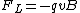Eq. (1), where q is the charge of the particles. This force deflects the particles so that a charge imbalance develops between opposite sides of the conductor. Deflection continues until the electric field Ey resulting from this charge imbalance produces a force Fy = qEy which cancels the Lorentz force. In practice, the equilibrium condition FL + Fy = 0 is achieved almost instantaneously, giving a steady-state Hall field as in Eq. (2). The current density is Jx = nqv, where n is the carrier density. The Hall resistivity, defined by Eq. (3), is thus given by Eq. (4). The Hall coefficient, defined by Eq. (5),
(2)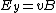(3)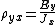(4)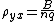(5)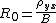satisfies Eq. (6)
(6)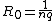and thus R0 provides a measure of the sign and magnitude of the mobile charge density in a conductor. Within the free-electron theory of simple metals, q is expected to be the electron charge -e, and n is taken to be n = ZnA, where Z is the valence of the metal and nA is the density of the atoms. This yields Eq. (7). (7)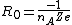See Free-electron theory of metals

Equation (7) is approximately valid in simple monovalent metals but fails drastically for other materials, often even giving the wrong sign. The explanation of the failures of Eq. (7) was one of the great early triumphs of the quantum theory of solids. The theory of band structure shows how collisions with the periodic array of atoms in a crystal can cause the current carriers to be holes which have an effective positive charge which changes the sign of the Hall coefficient. Band structure theory also accounts for the observed dependence of R0 on the orientation of the current and the magnetic field relative to the crystal axes, an effect which is very useful for studying the topology of the Fermi surface. See Band theory of solids, Fermi surface, Hole states in solids

In certain special field-effect transistors, it is possible to create an electron gas which is effectively two-dimensional. The Hall resistance for an idealized system in two dimensions is given by Eq. (8),

(8)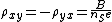where nS is the density of electrons per unit area (rather than volume). However, if the measured value of &rgr;xy for a high-quality (low-disorder) device is plotted as a function of B, the linear behavior predicted by Eq. (8) is observed only at low fields. At high fields the Hall resistance exhibits plateau regions in which it is a constant independent of B. Furthermore, the values of &rgr;xy on these plateaus are given quite accurately by the universal relation of Eq. (9),
(9)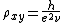where h is Planck's constant and &ngr; is an integer or simple rational fraction. The absolute accuracy with which Eq. (9) has been verified is better than 1 part in 106.

This extremely accurate quantization of &rgr;xy allows the realization of a new standard of resistance based solely on fundamental constants of nature. In addition, the quantum unit of Hall resistance, h/e2 &sime; 25,812.80 ohms, determines the fine-structure constant. See Electrical units and standards, Fundamental constants

The explanation of this remarkable phenomenon involves several subtle quantum-mechanical effects. In the quantum regime (small &ngr;), &rgr;xx, which is the dissipative (longitudinal) resistivity, approaches zero on the Hall plateaus. The quantization of the Hall resistance is intimately connected with this fact. It is speculated that at zero temperature the dissipation is zero and that Eq. (9) is then obeyed exactly. See Quantum mechanics

The nearly complete lack of dissipation in the quantum Hall regime is reminiscent of superconductivity. In both effects the ability of the current to flow without dissipation has its origin in the existence of a quantum-mechanical excitation gap, that is, a minimum threshold energy needed to disturb the special microscopic order in the system. See Entropy, Superconductivity

In the integer quantum Hall effect [where &ngr; in Eq. (9) in an integer], this excitation gap is a single-particle effect associated with the quantization by the strong magnetic field of the kinetic energy of the individual electrons into discrete states called Landau levels. In the fractional effect, the gap is associated with the highly collective, many-body ordering of the electrons into a quantum state which minimizes the strong Coulomb repulsion and hence lowers the overall energy. Thus, while the integer and fractional quantum Hall effects look superficially similar on a plot of resistivities versus magnetic field, their physical origins are actually quite different. See De Haas-van Alphen effect, Galvanomagnetic effects

McGraw-Hill Concise Encyclopedia of Physics. © 2002 by The McGraw-Hill Companies, Inc.
The following article is from The Great Soviet Encyclopedia (1979). It might be outdated or ideologically biased.

## Hall Effect

a phenomenon observed in a conductor that is carrying a current of density j and is placed in a magnetic field H. The Hall effect is the development of an electric field EH that is perpendicular to both H and j. The intensity of the electric field, which is called the Hall field, is equal to EH = RH j sin ±, where a is the angle between the vectors H and j (α < 180°). If H ± j, the intensity of the Hall field £H is maximum: £EH= RH j. The quantity R, which is known as the Hall factor, is the basic characteristic of the Hall effect. The effect was discovered in 1879 by E. H. Hall in strips of gold leaf.

To observe the Hall effect, a current is passed along the length of a rectangular plate consisting of the substance under investigation; the length I of the plate is considerably larger than its width b or thickness d (see Figure 1). The current I = jbd, and the magnetic field H is perpendicular to the surface of the plate. Electrodes are inserted in the middle of the sides of the plate that are perpendicular to the direction of current flow. The Hall electromotive force (emf) VH = E Hb = RHj/d is measured between the electrodes. Since the sense of the Hall emf is reversed when the direction of the magnetic field is reversed, the Hall effect is considered an odd galvanomagnetic phenomenon.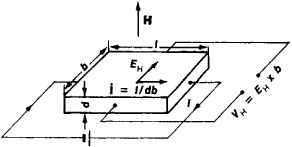Figure 1. The Hall effect

The simplest theory of the Hall effect attributes the development of the Hall emf to the interaction of charge carriers—that is, conduction electrons and holes—with the magnetic field. Under the influence of the electric field, the charge carriers drift with a drift velocity of vdr ≠ 0. The current density in the conductor j = neviv where n is the number of charge carriers per unit volume and e is the charge of each carrier. When the magnetic field is applied, each charge carrier experiences a Lorentz force F = e[Hvdr]. Under the influence of the Lorentz force, the particles are deflected in a direction that is perpendicular to both Vdr and H. As a result, a charge builds up on both sides of a conductor of finite size and an electrostatic field, namely, the Hall field, develops. In turn, the Hall field influences the charges and compensates the Lorentz force. Under equilibrium conditions, eEH = eHvdr and EH = (l/ne) Hj; hence R = l/ne cubic centimeters per coulomb (cm3/C). The sign of R is the same as the sign of the charge carriers. For metals, in which the concentration of carriers—that is, conduction electrons—is close to the number of atoms per unit volume (n ≈ 1022 cm”3), R ~ 10~3 cm3/C. In semiconductors, the concentration of carriers is substantially lower, and R ~ 105 cm3/C. The Hall factor R may be expressed in terms of the carrier mobility μ = eτ/m” and the electrical conductivity σ = j/E = envdr/E: R = μ/σ; here, m’ is the effective mass of a charge carrier and τ is the mean time between two successive collisions with scattering centers.

In descriptions of the Hall effect, the Hall angle is sometimes introduced. The Hall angle φ is the angle between the current j and the total electric field E: tg σ = EH/E = ωT, where ω is the cyclotron frequency of the charge carriers. In weak magnetic fields (ωT ≪ 1), the Hall angle φ ≈ ω may be regarded as the angle through which a moving charge is deflected over the time τ. This theory is valid for isotropic conductors (in particular, for polycrystals); for such conductors, m* and τ are constants. For isotropic semiconductors, the Hall factor is expressed in terms of the partial conductivities ae and <rh and of the electron concentration ne and the hole concentration nh. For weak magnetic fields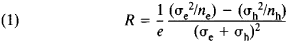For strong magnetic fields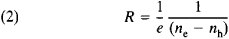When ne = nh = n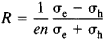for both weak and strong magnetic fields; the sign of R indicates whether n-type orp-type conductivity predominates.

For metals, the coefficient R depends on the band structure and on the shape of the Fermi surface. In the case of closed Fermi surfaces and in strong magnetic fields (ωτ ≫ 1), the Hall factor is isotropic and the expressions for R are the same as equation (2). For open Fermi surfaces, the factor R is anisotropic. However, if the direction of H with respect to the crystallographic axes is chosen so that no open sections of the Fermi surface arise, the expression for R is similar to equation (2).

In ferromagnetic materials, conduction electrons are influenced not only by the applied magnetic field but also by an intrinsic magnetic field: B = H + 4πM, where M is the magnetization. This situation gives rise to a peculiar Hall effect in ferromagnetic materials. Experiments have shown that EH = (RB + RaM, where R is the ordinary Hall factor and Ra is the extraordinary, or anomalous, Hall factor. A correlation has been established between Ra and the electrical resistivity of ferromagnetic materials.

Studies of the Hall effect have played an important role in the development of the band theory of solids. The Hail effect constitutes one of the most effective methods in use today for the study of the energy spectrum of charge carriers in metals and semiconductors. If R is known, the sign of charge carriers may be determined, the concentration of charge carriers may be estimated, and conclusions may often be reached as to the amount of impurities present in a substance, such as a semiconductor. The Hall effect also has a number of practical applications. It is used, for example, to measure the intensity of magnetic fields (seeMAGNETOMETER) and to amplify direct currents in analog computers. It is also employed in measurement technology as the basis of such devices as contactless ammeters (see).

### REFERENCES

Hall, E. H. “On the New Action of Magnetism on a Permanent Electric Current.” The Philosophical Magazine, 1880, vol. 10, p. 301.
Landau, L. D., and E. M. Lifshits. Elektrodinamika sploshnikh sred. Moscow, 1959.
Ziman, J. Elektrony i fonony: Teoriia iavlenii perenosa v tverdykh telakh. Moscow, 1962. (Translated from English.)
Weiss, H. Fizika gal’vanomagnitnykh poluprovodnikovykh priborov i ikh primenenie. Moscow, 1974. (Translated from German.)
Angrist, S. “Gal’vanomagnitnye i termomagnitnye iavleniia.” In the collection Nad chem dumaiut fiziki, fasc. 8: Fizika tverdogo tela: Elektronnye svoistva tverdogo tela. Moscow, 1972. Pages 45–55.

IU. P. GAIDUKOV

## Hall effect

[′hȯl i‚fekt]
(electromagnetism)
The development of a transverse electric field in a current-carrying conductor placed in a magnetic field; ordinarily the conductor is positioned so that the magnetic field is perpendicular to the direction of current flow and the electric field is perpendicular to both.
McGraw-Hill Dictionary of Scientific & Technical Terms, 6E, Copyright © 2003 by The McGraw-Hill Companies, Inc.
References in periodicals archive ?
Organisers: Petit Academy, Tatra Banka Foundation, Comenius University in Bratislava, Slovak University of Technology in Bratislava and SOVVA.Klaus von KlitzinProfessor Klaus von Klitzing is a German physicist, known for the discovery of the integer quantum Hall effect, for which he was awarded the 1985 Nobel Prize in Physics.
Their discovery of the magnetic spin Hall effect could lead to low-power, high-speed and high-capacity devices.
By placing together two specially designed 2D setups to study the quantum Hall effect, they were able to catch a glimpse of this fourth spatial dimension.
"Advancement in the sensor technologies is the key driver for the growth of Hall effect current sensor market"
German sensors specialist Novotechnik has responded to increasing demand for simple, flat, and affordable rotary sensors with the development of its RFD component series of non-contacting Hall effect devices.
The quantum Hall effect is simply a quantized form of the better known Hall effect.
Diodes Incorporated has introduced six high-performance Hall effect sensor switches, designed to save power in position and proximity detection roles in a variety of consumer, home appliance and industrial equipment.
Moore's Law may legislate electronics, but the 100-year-old plus Hall Effect technology is what inspired It electronics (ttelectronics.com) when designing its new steering sensor.
He along with others from the Hitachi Cambridge Laboratory and the Universities of Cambridge and Nottingham in the United Kingdom as well as the Academy of Sciences and Charles University in the Czech Republic, is the first to combine the spin-helix state and anomalous Hall effect to create a realistic spin-field-effect transistor (FET) operable at high temperatures, complete with an AND-gate logic device.
In this application, to get the data acquisition, Hall Effect sensor type a current transformer is connected to the primary side of the welding machine.
22 -- APEM Components introduces the IHS Hall Effect Pushbutton Switch, a contact less pushbutton switch that uses a magnet and a Hall Effect sensor, a transducer that varies its output voltage in response to changes in magnetic field.

Site: Follow: Share:
Open / Close